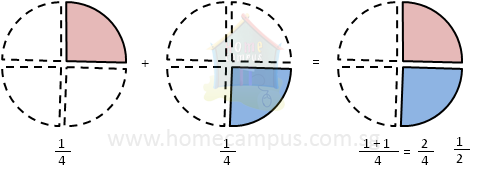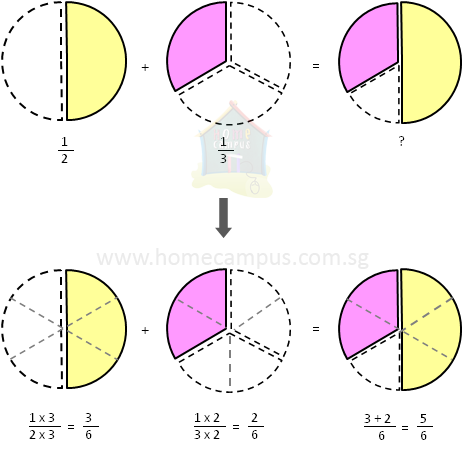## Adding Like and Unlike Fractions

Practice Unlimited Questions

#### What are like fractions?

Fractions with same denominators are called like fractions.

#### What are unlike fractions?

Fractions with different denominators are called unlike fractions.

#### How to add like fractions?

Step 1: Add all the numerators. (Denominator will remain the same.)
Step 2: Simplify if required.

See the example below

#### How to add unlike fractions?

Step 1: Change unlike fractions to like fractions. i.e. find a common denominator.
Step 2: Add all the numerators.
Step 3: Simplify if required.

See the example below

#### 1. The video below explains how to add proper fractions.

A proper fraction is a fraction in which the numerator is smaller than the denominator.

#### 2. Jen and Fred each had    1 4 of a pie. What fraction of the pie did they have together?

Jen  =>
 1 4

Fred  =>
 1 4

Jen  +  Fred  =>
 1 4
+
 1 41 4
+
 1 4

 1 + 1 4

 2 4

 2 / 2 4 / 2
(simplify)
 1 2

 1 2
a pie together.

#### 3. Charlie gives    1 2 of a pizza to Alfred and    1 3 of a pizza to Beatrice. How much of the pizza does Charlie give to Alfred and Beatrice together?

Alfred  =>
 1 2

Beatrice  =>
 1 3

Alfred  +  Beatrice  =>
 1 2
+
 1 31 2
+
 1 3

 1 x 3 2 x 3
+
 1 x 2 3 x 2

 3 6
+
 2 6
(changed the denominator to 6)
 5 6

Charlie gives
 5 6
of the pizza to Alfred and Beatrice together.
Since the two fractions,
 1 2
and
 1 3
, are unlike fractions (as the denominators are different), we must first change them to like fractions before we can add them (that is, find a common denominator).

In other words, at first, the pizzas are cut into different number of slices. To add them, we must cut them into same number of slices.